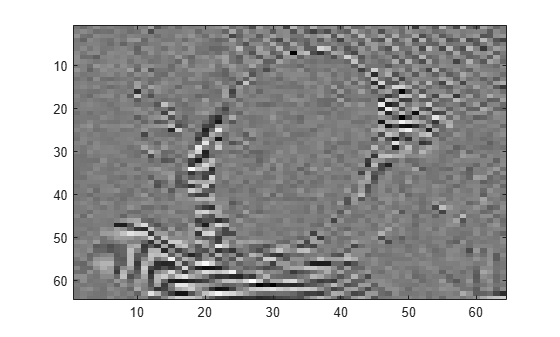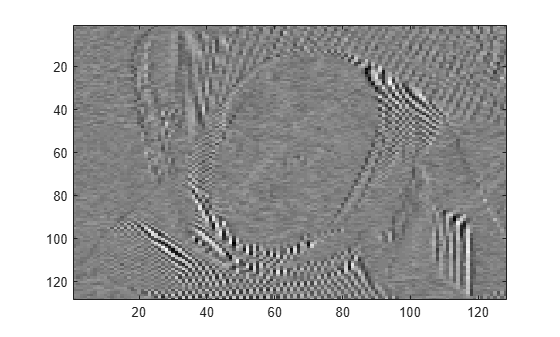# detcoef2

2-D detail coefficients

## Syntax

``y = detcoef2(o,c,s,n)``
``[h,v,d] = detcoef2('all',c,s,n)``
``y = detcoef2('compact',c,s,n)``

## Description

````y = detcoef2(o,c,s,n)` extracts from the wavelet decomposition structure `[c,s]` the detail coefficients of orientation `o` at level `n`. For more information on `c` and `s`, see `wavedec2`.```

example

````[h,v,d] = detcoef2('all',c,s,n)` returns the horizontal `h`, vertical `v`, and diagonal `d` detail coefficients at level `n`.`detcoef2('a',c,s,n)` is equivalent to `detcoef2('all',c,s,n)`.```
````y = detcoef2('compact',c,s,n)` returns all the detail coefficients stored row-wise.`detcoef2('c',c,s,n)` is equivalent to `detcoef2('compact',c,s,n)`.If `[H,V,D] = detcoef2('all',c,s,N)` and `Y = detcoef2('compact',c,s,N)`, then `Y = [H(:)' V(:)' D(:)']`.```

## Examples

collapse all

This example shows how to extract detail coefficients from a discrete wavelet analysis of an image. This example uses zero-padding.

```origmode = dwtmode('status','nodisplay'); dwtmode('zpd','nodisplay'); load woman imagesc(X) colormap(gray)```Obtain the wavelet decomposition of the image down to level two using the Haar wavelet.

```[c,s] = wavedec2(X,2,'haar'); size(X)```
```ans = 1×2 256 256 ```
`size(c)`
```ans = 1×2 1 65536 ```
`s`
```s = 4×2 64 64 64 64 128 128 256 256 ```

Extract the detail coefficients at level 2 in each orientation from the wavelet decomposition structure `[c,s]`. Display the diagonal detail coefficients.

```[chd2,cvd2,cdd2] = detcoef2('all',c,s,2); size(cdd2)```
```ans = 1×2 64 64 ```
```imagesc(cdd2) colormap(gray)```Extract the detail coefficients at level 1 in each orientation. Display the vertical detail coefficients.

```[chd1,cvd1,cdd1] = detcoef2('all',c,s,1); size(cvd1)```
```ans = 1×2 128 128 ```
```imagesc(cvd1) colormap(gray)```Restore the original extension mode.

`dwtmode(origmode,'nodisplay')`

## Input Arguments

collapse all

Orientation of detail coefficients, specified as:

• `'h'` — Horizontal

• `'v'` — Vertical

• `'d'` — Diagonal

Wavelet decomposition vector, specified as a real-valued vector. The vector `c` contains the approximation and detail coefficients organized by level. The bookkeeping matrix `s` is used to parse `c`. See `wavedec2`.

Data Types: `double`

Bookkeeping matrix, specified as an integer-valued matrix. The matrix `s` contains the dimensions of the wavelet coefficients by level and is used to parse the wavelet decomposition vector `c`. See `wavedec2`.

Data Types: `double`

Detail level to extract from the wavelet decomposition, specified as an integer. The integer `n` must be in the interval `[1,size(s,1)-2]`.

## Output Arguments

collapse all

Detail coefficients, returned as a vector or matrix.

Data Types: `double`

Horizontal detail coefficients, returned as a matrix.

Data Types: `double`

Vertical detail coefficients, returned as a matrix.

Data Types: `double`

Diagonal detail coefficients, returned as a matrix.

Data Types: `double`

## Version History

Introduced before R2006a